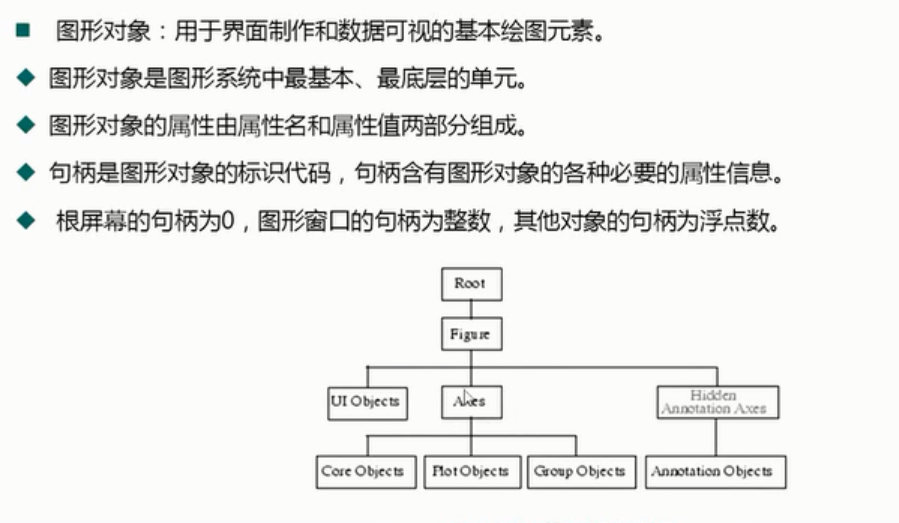## 《MATLAB》小常识_matlab中target函数用法-程序员宅基地

#### 3、setdiff(A,B);返回在A中有，而B中没有的值，结果向量将以升序排序返回

a=setdiff(target,s,'stable');%返回在target中有，而s中没有的值，顺序不变

setdiff还可以带有参数'stable'或者'sorted'。

'stable'表示差集中元素的顺序是按照A中原有相对顺序。

'sorted'表示差集中元素的顺序是排序后的

#### 4、已知变量 x1 x2 x3 ... x100  和 100*100的矩阵 A。如何实现 xi=A(:,i) i=1,2,3...100？

x = mat2cell(A,100,ones(100,1));%x{1}就是x1

1. ``````A=rand(100);
x=cell(1,100);
n=size(A,1);
for i=1:n
x{i}=A(:,i);
end    ``````
用x{1}……x{100}分别表示你的x1……x100

x{1}
0.8147
0.9058
0.1270
0.9134
0.6324
0.0975
0.2785
0.5469
0.9575
0.9649……

#### 5、matlab中数组倒序：

inv(A)逆
filplr(A)左右翻转
flipud(A)上下翻转
rot90(A)逆时针转90度
rot90(A,2)转180度
rot90(A,-1)顺时针转90度

#### 6、matlab取商、取模运算

matlab 求商，余数的方法 - 咆哮的狼 - 程序员宅基地  https://blog.csdn.net/lsg32/article/details/8753668

【转】取模（mod）与取余（rem）的区别——Matlab学习笔记 - 编著人 - 博客园  https://www.cnblogs.com/xfzhang/archive/2010/11/25/1887214.html

#### 7、图像对象和句柄``````%% V. 图像对象和句柄
%%
% 1. 如何设置线条的属性呢？
x = 0:0.01:2*pi;
y = sin(x);
h = plot(x,y);
grid on
get(h)
set(h,'linestyle','-','linewidth',5,'color','k')

%%
% 2. 如何修改网格的间隔呢？
set(gca,'xtick',0:0.5:7)%gca：get current axis 获得当前坐标轴
set(gca,'ytick',-1:0.1:1)

%%
% 3. 如何设置图例的字体及大小呢？
x = 0:0.01:2*pi;
y1 = sin(x);
y2 = cos(x);
plot(x,y1,'r')
hold on
plot(x,y2,'-.b')
h = legend('sin(x)','cos(x)');
set(h,'fontsize',16,'color','k','edgecolor','r','textcolor','w')

%%
% 4. 如何拆分图例呢？
x = 0:0.01:2*pi;
y1 = sin(x);
y2 = cos(x);
h1 = plot(x,y1,'r');
hold on
h2 = plot(x,y2,'-.b');
ax1 = axes('position',get(gca,'position'),'visible','off');
legend(ax1,h1,'sin(x)','location','northwest')
ax2 = axes('position',get(gca,'position'),'visible','off');
legend(ax2,h2,'cos(x)','location','northeast')
``````

#### 8、使用变量前，预分配内存空间

clear all
clc
n = 30000;
tic;
for k = 1:n
a(k) = 1;
end
time = toc;
disp(['未预分配内存下动态赋值长为',num2str(n),'的数组时间是:',num2str(time),'秒！'])

分配内存如下：

tic
b = zeros(1,n);
for k = 1:n
b(k) = 1;
end
time = toc;
disp(['预分配内存下动态赋值长为',num2str(n),'的数组时间是:',num2str(time),'秒！'])

#### 9、matlab里面的计时函数：

Matlab7的计时函数主要有tic,toc,cputime和etime等，计时函数可以定量的计算完成制定程序所消耗的时间资源，因为可以作为比较程序优劣的一个重要标准。

a、tic和toc函数

这两个函数一般配合使用，tic表示计时的开始，toc表示计时的结束。

格式如：

tic

任意表达式

toc

t=toc

b、cputime函数

cputime函数返回从调用该函数起所用的总的Cpu时间，单位以秒计算。

格式如：

t=cputime;

任意表达式或者程序

e=cputime-t;

c、etime函数

e=etime(t2,t1)命令返回向量t1和t2之间的时间段，t1和t2必须含有由clock函数返回的6个元素，即[Year Month Day Hour                    Minute Second]。

举例说明：

>> x=rand(2048,1);

>> t=clock;

>> fift(x);

>> etime(clock,t);

ans=

14.53

edit mean

edit newff

### 自己手动实现dubbo-程序员宅基地

1.大概花了很多周的时间上班的同时实现了dubbo的基础功能。下面介绍下大致技术。rpc实现技术：通过netty实现，序列化是Hessian ，动态类技术是javassist,同时把dubbo源码里面的ClassGenerator 类拿过来。整合spring：自定义spring标签。监听spring的DisposableBean，BeanNameAware，ApplicationContextA

### Nginx：影响高并发性能优化的主要参数配置（含Nginx运维标准文件）_worker_processes 6;-程序员宅基地

Nginx是一个高性能的HTTP和反向代理web服务器，在连接高并发的情况下，Nginx是Apache服务不错的替代品。_worker_processes 6;

### 我理解的冒泡排序_理解 冒泡排序-程序员宅基地

package demo09;import java.util.Arrays;/** * @Author: micro cloud fly * @Description: 冒泡排序 * @Date: Created in 4:39 下午 2020/9/27 */public class Test { public static void main(String[] args) { int[] arr = new int[]{ 1,._理解 冒泡排序